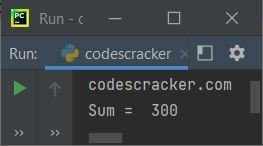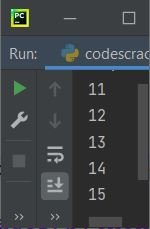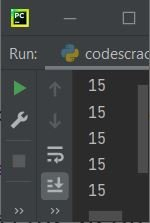# Python Identifier (with Example)

As the name suggests, an identifier is a name that identifies an object in a Python program. An object may be a variable, class, function, etc. For example:

```var = "codescracker.com"
a = 100
b = 200

def myfun():
print(var)

class myclass:
def sum(self, num_one, num_two):
return num_one+num_two

myfun()
obj = myclass()
print("Sum = ", obj.sum(a, b))```

In the above program, the list of identifiers is as follows:

• var
• a
• b
• myfun
• myclass
• sum
• num_one
• num_two
• obj

where myfun and sum are the two identifiers used to name functions, myclass is another identifier used to name classes, and all others are used to name variables. Basically, identifiers are the building blocks of a Python program. The output of the preceding program should look exactly like this:Don't worry about the code; just look at the identifiers. In the following chapters, you will learn about functions, classes, and so on.

Important: Identifiers should not be keywords.

Note: Python is a case-sensitive programming language. Case-sensitive means num, Num, NUM, nUm, and nuM are all different variables or identifiers.

### How to Name an Identifier in Python

In Python, we can use a combination of letters (a-z, A-Z), numbers (0-9) and underscore (_) to name an identifier. For example, codescracker, codes_cracker, codes12cracker, codes123, etc.

## Rules for Naming Identifiers in Python

Here is the list of rules that must be followed when naming or creating a variable, class, function, etc. in Python:

• Or identifiers cannot start with any character other than A-Z, a-z, or _ (underscore).
• After A-Z, a-z, or _, you can use any combination of A-Z, a-z, 0-9, and _.
• Identifiers in Python cannot be named using Python's keyword.
• So, I recommend you see the list of Python keywords before naming an identifier.

## Python Identifiers Example

Let's create a program in Python to prove that Python is a case-sensitive language. That is, two identifiers, say num and Num, get treated as two different identifiers by the compiler:

```num = 11
Num = 12
NUM = 13
nUm = 14
nuM = 15

print(num)
print(Num)
print(NUM)
print(nUm)
print(nuM)```

The snapshot given below shows the exact output produced by the above Python program:You see! All the identifiers print different values, but the name is the same if their case gets ignored. If Python is not a case-sensitive language, then the output should be 15, five times. That is, if you replace the above program with the one given below:

```num = 11
num = 12
num = 13
num = 14
num = 15

print(num)
print(num)
print(num)
print(num)
print(num)```

Then the output must be equal to the snapshot given below:Python Online Test

« Previous Tutorial Next Tutorial »